# EXERCISE – 7.2

1. Which congruence criterion do you use in the following?

(a) Given: AC = DF

AB = DE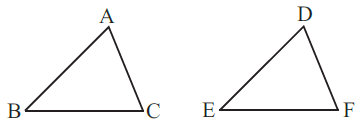BC = EF

So, ΔABC ΔDEF

Solution:-

By SSS congruence property:- Two triangles are congruent if the three sides of one triangle are respectively equal to the three sides of the other triangle.

ΔABC ≅ ΔDEF

(b) Given: ZX = RP

RQ = ZY

PRQ = XZY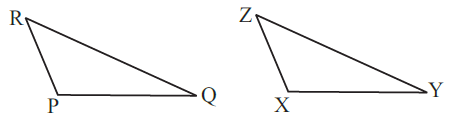Solution:-

By SAS congruence

property:- Two triangles are congruent if the two sides and the included angle of one are respectively equal to the two sides and the included angle of the other.

ΔACB ≅ ΔDEF

(c) Given: MLN = FGH

NML = GFH

ML = FG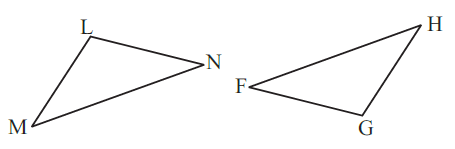So, ΔLMN ΔGFH

Solution:-

By ASA congruence property:- Two triangles are congruent if the two angles and the included side of one are respectively equal to the two angles and the included side of the other.

ΔLMN ≅ ΔGFH

(d) Given: EB = DB

AE = BC

A = C = 90o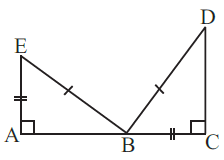So, ΔABE ΔACD

Solution:-

By RHS congruence property:- Two right triangles are congruent if the hypotenuse and one side of the first triangle are respectively equal to the hypotenuse and one side of the second.

ΔABE ≅ ΔACD

1. You want to show that ΔART ΔPEN,

(a) If you have to use SSS criterion, then you need to show

(i) AR = (ii) RT = (iii) AT =

Solution:-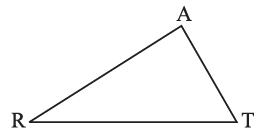We know that,

SSS criterion is defined as, two triangles are congruent if the three sides of one triangle are respectively equal to the three sides of the other triangle.

∴ (i) AR = PE

(ii) RT = EN

(iii) AT = PN

(b) If it is given that T = N and you are to use SAS criterion, you need to have

(i) RT = and (ii) PN =

Solution:-

We know that,

SAS criterion is defined as, two triangles are congruentif the two sides and the included angle of one are respectively equal to the two sides and the included angle of the other.

∴ (i) RT = EN

(ii) PN = AT

(c) If it is given that AT = PN and you are to use ASA criterion, you need to have

(i) ? (ii) ?

Solution:-

We know that,

ASA criterion is defined as, two triangles are congruent if the two angles and the included side of one are respectively equal to the two angles and the included side of the other.

Then,

(i) ∠ATR = ∠PNE

(ii) ∠RAT = ∠EPN

1. You have to show that ΔAMP ΔAMQ.

In the following proof, supply the missing reasons.

 Steps Reasons (i) PM = QM (i) … (ii) ∠PMA = ∠QMA (ii) … (iii) AM = AM (iii) … (iv) ΔAMP ≅ ΔAMQ (iv) …

Solution:-

 Steps Reasons (i) PM = QM (i) From the given figure (ii) ∠PMA = ∠QMA (ii) From the given figure (iii) AM = AM (iii) Common side for the both triangles (iv) ΔAMP ≅ ΔAMQ (iv) By SAS congruence property:- Two triangles are congruent if the two sides and the included angle of one are respectively equal to the two sides and the included angle of the other.
1. In ΔABC, A = 30o, B = 40oand C = 110o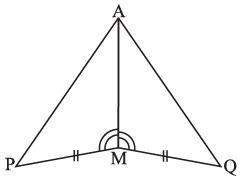In ΔPQR, P = 30o, Q = 40o and R = 110o

A student says that ΔABC ΔPQR by AAA congruence criterion. Is he justified? Why or Why not?

Solution:-

No, because the two triangles with equal corresponding angles need not be congruent. In such a correspondence, one of them can be enlarged copy of the other.

1. In the figure, the two triangles are congruent. The corresponding parts are marked. We can write ΔRAT ?

Solution:-

From the given figure,

We may observe that,

∠TRA = ∠OWN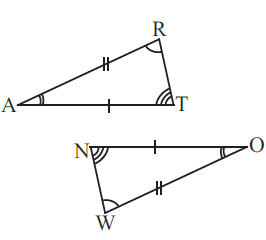∠TAR = ∠NOW

∠ATR = ∠ONW

Hence, ΔRAT ≅ ΔWON

1. Complete the congruence statement:

ΔBCA ΔQRS

Solution:-

First consider the ΔBCA and ΔBTA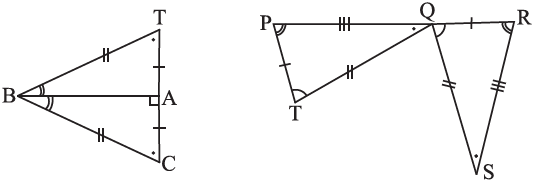From the figure, it is given that,

BT = BC

Then,

BA is common side for the ΔBCA and ΔBTA

Hence, ΔBCA ≅ ΔBTA

Similarly,

Consider the ΔQRS and ΔTPQ

From the figure, it is given that

PT = QR

TQ = QS

PQ = RS

Hence, ΔQRS ≅ ΔTPQ

1. In a squared sheet, draw two triangles of equal areas such that

(i) The triangles are congruent.

(ii) The triangles are not congruent.

What can you say about their perimeters?

Solution:-

(ii)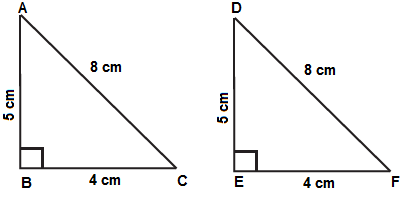In the above figure, ΔABC and ΔDEF have equal areas.

And also, ΔABC ≅ ΔDEF

So, we can say that perimeters of ΔABC and ΔDEF are equal.

(ii)

In the above figure, ΔLMN and ΔOPQ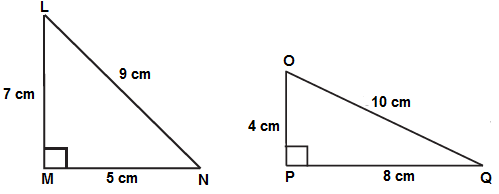ΔLMN is not congruent to ΔOPQ

So, we can also say that their perimeters are not same.

1. Draw a rough sketch of two triangles such that they have five pairs of congruent parts but still the triangles are not congruent.

Solution:-

Let us draw triangles LMN and FGH.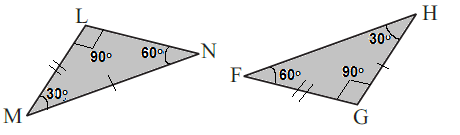In the above figure, all angles of two triangles are equal. But, out of three sides only two sides are equal.

Hence, ΔLMN is not congruent to ΔFGH.

1. If ΔABC and ΔPQR are to be congruent, name one additional pair of corresponding parts. What criterion did you use?

Solution:-

By observing the given figure, we can say that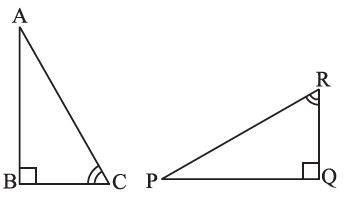∠ABC = ∠PQR

∠BCA = ∠PRQ

The other additional pair of corresponding part is BC = QR

∴ ΔABC ≅ ΔPQR

1. Explain, why ΔABC ΔFED

Solution:-

From the figure, it is given that,∠ABC = ∠DEF = 90o

∠BAC = ∠DFE

BC = DE

By ASA congruence property, two triangles are congruent if the two angles and the included side of one are respectively equal to the two angles and the included side of the other.

ΔABC ≅